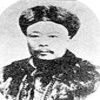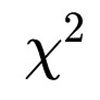#### You may also like### Very Old Man

Is the age of this very old man statistically believable?### Reaction Timer Timer

How can you time the reaction timer?### Chi-squared Faker

How would you massage the data in this Chi-squared test to both accept and reject the hypothesis?

# Hypothetical Shorts

### Why do this problem?

This task will challenge students to think about the meaning of hypothesis tests and challenge some common misconceptions about them.  Hypothesis tests are used (and misused) in many areas of science and social science, so becoming aware of these issues at an early stage will help to protect them from falling into serious traps later on.  The questions are grouped thematically.

### Possible approach

You may wish to use the problem Stats Statements before this one, which is about probability and statistics more generally.  You may also want to look at some or all of Robin's Hypothesis Testing, Powerful Hypothesis Testing or What is a Hypothesis Test? before or after working on this problem.  You might also have an initial discussion about these questions, then work on some or all of the other resources, and return to these questions afterwards.  In that way, students' minds will be more attuned to some of the issues involved as they think more deeply about hypothesis testing.

This problem is very well suited to discussion. As there are no calculations involved (at least superficially), it is very easy for all students to get into this problem at a level which suits them. One approach might be to ask for an immediate, instinctive response to the questions before asking them to assess them in more detail. What factors do they need to take into account in order to answer the questions? Does it depend on the specific hypothesis test? Might there be any exceptions? And then to reflect: were their gut-feelings right or wrong? Were there any surprises?  (Note that question 5 is certainly true if question 4 is true, but they might both be false.)

This problem offers a good chance to practise explaining complicated ideas in statistics.  Students could try to explain their thoughts verbally to each other.  Giving a good explanation requires a sound analysis of the statistics.  Does the audience think that the explanation is sound or convincing?

This problem gives an opportunity to discuss the idea of conditional (if ... then ...) statements, as several of the questions use them.  It also provides a context in which to explore the power of counterexamples: for example, constructing a single example in which 'the p-value is less than 0.05, but the alternative hypothesis is false' would show that the statement 'If the p-value is less than 0.05, then the alternative hypothesis is true' cannot be true.

It is important to note that this problem is likely to raise many questions about hypothesis testing. An exploration of such questions will lead to a stronger understanding of this important area of statistics, which can only impact positively on students' future use of this material in their school studies and beyond.  Many of the questions are addressed, directly or indirectly, in the other resources mentioned above.

### Key questions

This problem may feel very easy to some students, who might just give an "obvious" answer, while others might find some of the questions too sophisticated to tackle.  To encourage students to think their way through each statement, you could pose questions such as:

• Can you give a concrete example of a hypothesis test?
• What might happen in this test?
• What if the null hypothesis is actually true/false - what might happen then?

### Possible extension

Students could be asked how we could do further experiments (questions 7 and 8) while still obtaining valid results.  This question has a qualitative answer, but can also (with more sophistication) be answered quantitatively.

### Possible support

Students could use the interactivities in Robin's Hypothesis Testing or Powerful Hypothesis Testing to gain a sense of what p-values mean in a hypothesis test.  In this way, they can control the parameters of an experiment and observe the results, repeating as many times as they need to in order to give meaningful answers to the questions.

If students need support with understanding conditional statements, they could explore Iffy Logic.